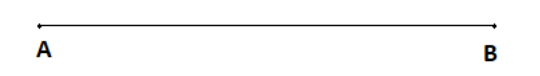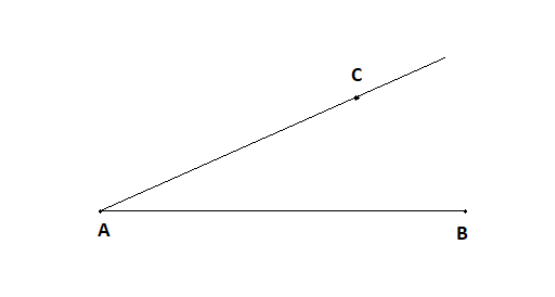Courses
Courses for Kids
Free study material
Offline Centres
MoreLast updated date: 06th Dec 2023
Total views: 282.3k
Views today: 4.82k

# Construct an angle measuring $35^\circ$ using protractor.Verified
282.3k+ views
Hint: We have to construct an angle measuring $35^\circ$ using protractor. For that, first of all draw a horizontal line segment of any length you want. Name the end points of this line segment A and B. Now, place the midpoint of the protractor at point A and mark a point at $35^\circ$. Now, join the point with vertex A and we will get our angle.

In this question, we have to construct an angle measuring $35^\circ$ using the protractor.
Steps for constructing an angle using protractor:
Step 1:
First of all, we need to construct a base. So, draw a horizontal line segment of any measure you wish.
Name these endpoints of the line segment A and B.Step 2:
Now, we have to construct an angle $\angle A = 35^\circ$.
For that, place the midpoint of the protractor at point A and then mark a point at angle 35.
Now, join that point to the vertex A.Hence, we have constructed an angle $\angle CAB = 35^\circ$.

Note: In Euclidean geometry, an angle is the figure formed by two rays, called the sides of the angle, sharing a common endpoint, called the vertex of the angle. Angles formed by two rays lie in the plane that contains the rays.